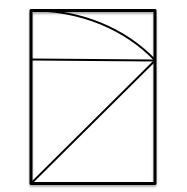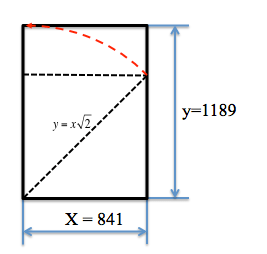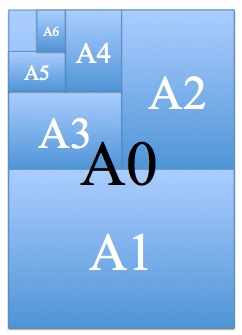# Drawing formats standardizedThe paper supports used for the representation of engineering objects have dimensions that are accepted internationally widespread, or what is the same, found “standardized”.

ISO 5457 specifies the characteristics of the formats. This standard adopts one or several different designations (can be divided into several standards) in the adaptations that take place in each country.

For example, in Spain, we UNE 1–026–83/2, UNE EN ISO 216 …

These dimensions are given under the concept of “drawing formats” and are obtained by applying three rules:

• Reference: The basic format, called A0, has a square meter. ( x * y = 1 square meter)
• Similarity: The relationship between the sides of the form (base / height) is between the value of the side of a square and the length of its diagonal (1/Following two).
• Bent: When bending a format for half its longest side, you get two identical formats (following format)So, if we double A0 format, A1 provides two formats. If we double A1 format, A2 produces two formats. If we double A2 format, A3 provides two formats …We see therefore that an A0 format can be obtained by bending:

• of A1 ( 2 elevated 1 )
• four A2 ( 2 elevated 2 )
• ocho A3 ( 2 elevated 3 )
• 2 N AN elevated formats